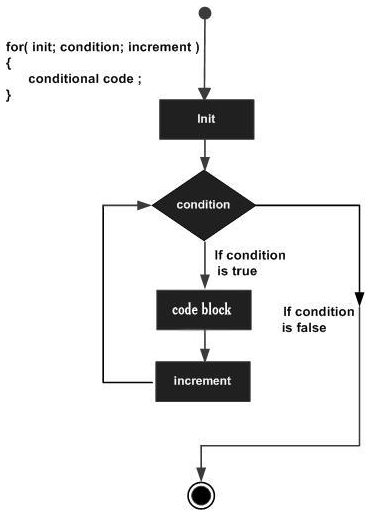# Java for loop

A for loop is a repetition control structure that allows you to efficiently write a loop that needs to be executed a specific number of times.

A for loop is useful when you know how many times a task is to be repeated.

## Syntax

The syntax of a for loop is −

for(initialization; Boolean_expression; update) {
// Statements
}

Here is the flow of control in a for loop −

• The initialization step is executed first, and only once. This step allows you to declare and initialize any loop control variables and this step ends with a semicolon (;).

• Next, the Boolean expression is evaluated. If it is true, the body of the loop is executed. If it is false, the body of the loop will not be executed and control jumps to the next statement past them for a loop.

• After the body of the for loop gets executed, the control jumps back up to the update statement. This statement allows you to update any loop control variables. This statement can be left blank with a semicolon at the end.

• The Boolean expression is now evaluated again. If it is true, the loop executes and the process repeats (body of the loop, then update step, then Boolean expression). After the Boolean expression is false, the for loop terminates.

## Flow Diagram## Example

Following is an example code of the for loop in Java.

Live Demo

public class Test {
public static void main(String args[]) {
for(int x = 10; x < 20; x = x + 1) {
System.out.print("value of x : " + x );
System.out.print("");
}
}
}


This will produce the following result −

## Output

value of x : 10
value of x : 11
value of x : 12
value of x : 13
value of x : 14
value of x : 15
value of x : 16
value of x : 17
value of x : 18
value of x : 19